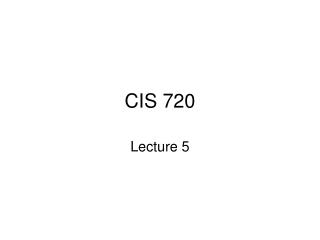DownloadDownload PresentationCIS 720

# CIS 720

Télécharger la présentation## CIS 720

- - - - - - - - - - - - - - - - - - - - - - - - - - - E N D - - - - - - - - - - - - - - - - - - - - - - - - - - -
##### Presentation Transcript

1. CIS 720 Lecture 5

2. Techniques to avoid interference • Disjoint variables • If the write set of each process is disjoint from the read and write set of other processes, then the proof outlines will be interference free.

3. Avoiding interference • Weakened assertions x= 0; co x = x + 1 // x = x + 2 od

4. Avoiding interference • Global Invariants: Assume that every assertion in the proof outline of Pi is of the form I /\ L, where L refers to local variables of Pi or to variables that only Pi writes. - Then the proof outlines will be interference free.

5. x = 0 ; y = 0; z = 0 co x = 1 y := 1 // if y = 1  z = 1 oc

6. Avoiding interference • Synchronization • Await Statement rule {P /\ B } S {Q} {P} < await(B)  S > {Q}

7. b = false x = 0 ; y = 0; z = 0 co x = x + 1 b := true z = x + 3 // < await b  y = x > oc

8. Bank transactions total = 0; i = 0; co // Transfer(x,y,am): Auditor: < await( (i < x /\ i < y) \/ {A1: Total = ac+….+ac[i-1] } (i > x /\ i > y)) do i < n  ac[x] = ac[x] – am; i = i + 1 ac[y] = ac[y] + am > total = total + ac[i] od oc

9. Safety and liveness properties • A safety property states that something bad will not happen • A liveness property states that something good will eventually happen

10. Proving safety property • BAD= predicate characterizing the bad property • GOOD = not BAD • Prove that GOOD is an invariant

11. Liveness property • A statement is eligible if it is the next action that could be executed • Scheduling policy determines the next statement to be executed

12. Unconditional fairness: A scheduling policy is unconditionally fair if every unconditional atomic action (one which does not have a guard) that is eligible is executed eventually. • Weak Fairness: A scheduling policy is weak fair if • It is unconditionally fair • Every conditional action that is eligible is eventually executed assuming that its guard becomes true and remains true.

13. Strong Fairness: A scheduling policy is strong fair if • It is unconditionally fair • Every conditional action that is eligible is eventually executed assuming that its guard becomes true and becomes true infinitely often

14. Critical Section problem • Process i do (true) entry protocol; critical section; exit protocol; non-critical section od

15. Correctness • Mutual exclusion: at most one process at a time is executing its critical section • Absence of deadlock: If two or more processes are trying to enter their critical section, at least one will succeed • Absence of unnecessary delay: If a process is trying to enter its critical section and the other processes are executing their non-critical sections or have terminated then the first process is not prevented from entering its critical section. • Eventual entry: A process that is attempting to enter its critical section will eventually succeed.

16. Invariant based approach CS1 CS2 do (true) do (true)  entry protocol; entry protocol; in1 = true in2 = true critical section critical section exit protocol; exit protocol; in1 = false in2 = false non-critical section non-critical section od

17. Invariant based approach CS1 CS2 do (true) do (true)  <await (!in2)  in1 = true> <await(!in1)  in2 = true> critical section critical section in1 = false in2 = false non-critical section non-critical section od

18. Invariant based approach CS1 CS2 do (true) do (true)  <await (!lock)  lock = true> <await(!lock)  lock = true> critical section critical section lock = false lock = false non-critical section non-critical section od

19. Test and set instruction CS1 CS2 do (true) do (true)  while (TS(lock)) skip; while(TS(lock)); critical section critical section lock = false lock = false non-critical section non-critical section od

20. Implementing await statements • CSenter while (!B) { CSexit; CSenter } S; CSexit